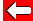# More Quadratic Equations

Let's now look at equations of the form ax² + bx = 0
e.g. x² + 2x = 0

If you know how to factorise, you'll be fine with this. We factorise the equation into:
x (x + 2) = 0

This could be true when x = 0, since 0 times the bracketed term = 0. However, there remains another possibility: the bracketed term itself is 0:
(x + 2) = 0

We can now ignore the brackets: x + 2 = 0
So the solution is: x = -2

So, the solutions to x² + 2x = 0 are x = 0 or -2Go back a pageMaths MenuGo to next page